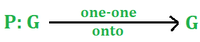# Permutation Groups and Multiplication of Permutation

• Difficulty Level : Basic
• Last Updated : 18 May, 2022

Let G be a non-empty set, then a one-one onto mapping to itself that is as shown below is called a permutation.• The number of elements in finite set G is called the degree of Permutation.
• Let G have n elements then Pn is called a set of all permutations of degree n.
• Pn  is also called the Symmetric group of degree n.
• Pn is also denoted by Sn.
• The number of elements in Pn or Sn isExamples:

Case1: Let G={ 1 } element then permutation are Sn or PnCase 2: Let G= { 1, 2 } elements then permutations areCase 3: Let G={ 1, 2, 3 } elements then permutation are 3!=6. These are,Reading the Symbol of Permutation

Suppose that a permutation is• First, we see that in a small bracket there are two rows written, these two rows have numbers. The smallest number is 1 and the largest number is 6.
• Starting from the left side of the first row we read as an image of 1 is 2, an image of 1 is 2, an image of 2 is 3, an image of 3 is 1, an image of 4 is 4 (Self image=identical=identity), an image of 5 is 6 and image of 6 is 5.
• The above thing can be also read as: Starting from the left side of the first row 1 goes to 2, 2goes to 3, 3goes to,4 goes to 4,5 goes to 6, and 6 goes to 5.

A cycle of length 2 is called a permutation.

Example:

1)Length is 2, so it is a transposition.

2)Length is three, so it is not a transposition.

Multiplication of Permutation

Problem: IfFind the product of permutation A.B and B.A

Solution:Here we can see that in first bracket 1 goes to 2 i.e. image of 1 is 2, and in second row 2 goes to 3 i.e. image of 2 is 3.

Hence, we will write 3 under 1 in the bracket shown below,Do above step with all elements of first row, answer will beSimilarly,My Personal Notes arrow_drop_up